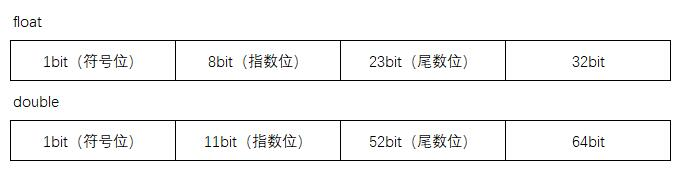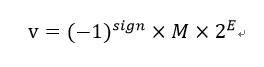# 1 浮点计算问题

``````
public class Oct2Bin {
public static void main(String[] args) {
double a = 0.1d;
double b = 0.2d;
System.out.println(a + b);
}
}

``````

# 2 小数计算丢精度原因

### 2.2 、0.3背后丢精度的原因

``````
0.3 x 2 = 0.6 ------取整数部分 0

0.6 x 2 = 1.2 ------取整数部分 1

0.2 x 2 = 0.4 ------取整数部分 0

0.4 x 2 = 0.8 ------取整数部分 0

0.8 x 2 = 1.6 ------取整数部分 1

0.6 x 2 = 1.2 ------取整数部分 1

0.2 x 2 = 0.4 ------取整数部分 0

0.4 x 2 = 0.8 ------取整数部分 0

0.8 x 2 = 1.6 ------取整数部分 1

……. 无限循环..........循环体1001

``````

# 3 Java中的float存储方式及计算方法

### 3.1 float的存储方式float在内存中共占32位，double共占64位，这也是单精度和双精度浮点数名称的由来。 在IEEE 754标准中，严格的表示形式是这样的：### 3.2 举例论证数据存储

``````
520 = 5.20 * 10 ^ (2)

``````

8.25 1000.01

``````
1000.01 = (-1)^0 * 1.00001 * 2 ^ (3)

``````

``````

0 10000010 00000000000000000100001

``````

-----------------------